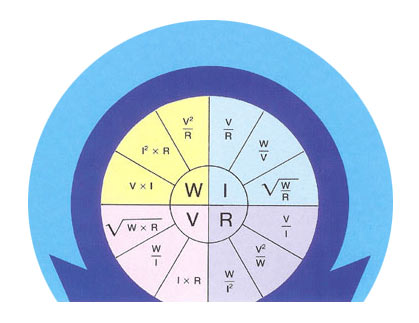## 联系信息# Ohms Law

The electrical resistance required at various wattages and voltages may be calculated using the following formulae, as may wattage, voltage and current values.

Where:

 V Voltage (Volts) W Power (Watts) I Current (Amps) R Resistance at 20°C (ohms)